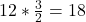2. Given the following equation: 2 KCIO3 => 2 KCl + 3 O2 How many moles of O2 can be produced by letting 12.00 moles of KClO3 rea

Question

2. Given the following equation: 2 KCIO3 —> 2 KCl + 3 O2
How many moles of O2 can be produced by letting 12.00 moles of KClO3 react?

show work. helppp asap

in progress 0
5 months 2021-08-27T15:17:59+00:00 1 Answers 0 views 0

18 moles of O2 can be produced by letting 12.00 moles of KClO3 react

Explanation:

The balanced equation is

2KCIO3 —> 2 KCl + 3 O2

As per the reaction,

2 mole of KClO3 produces 3 mole of O2 gas

Hence, 12 moles of KClO3 will produceHence,   18 moles of O2 can be produced by letting 12.00 moles of KClO3 react